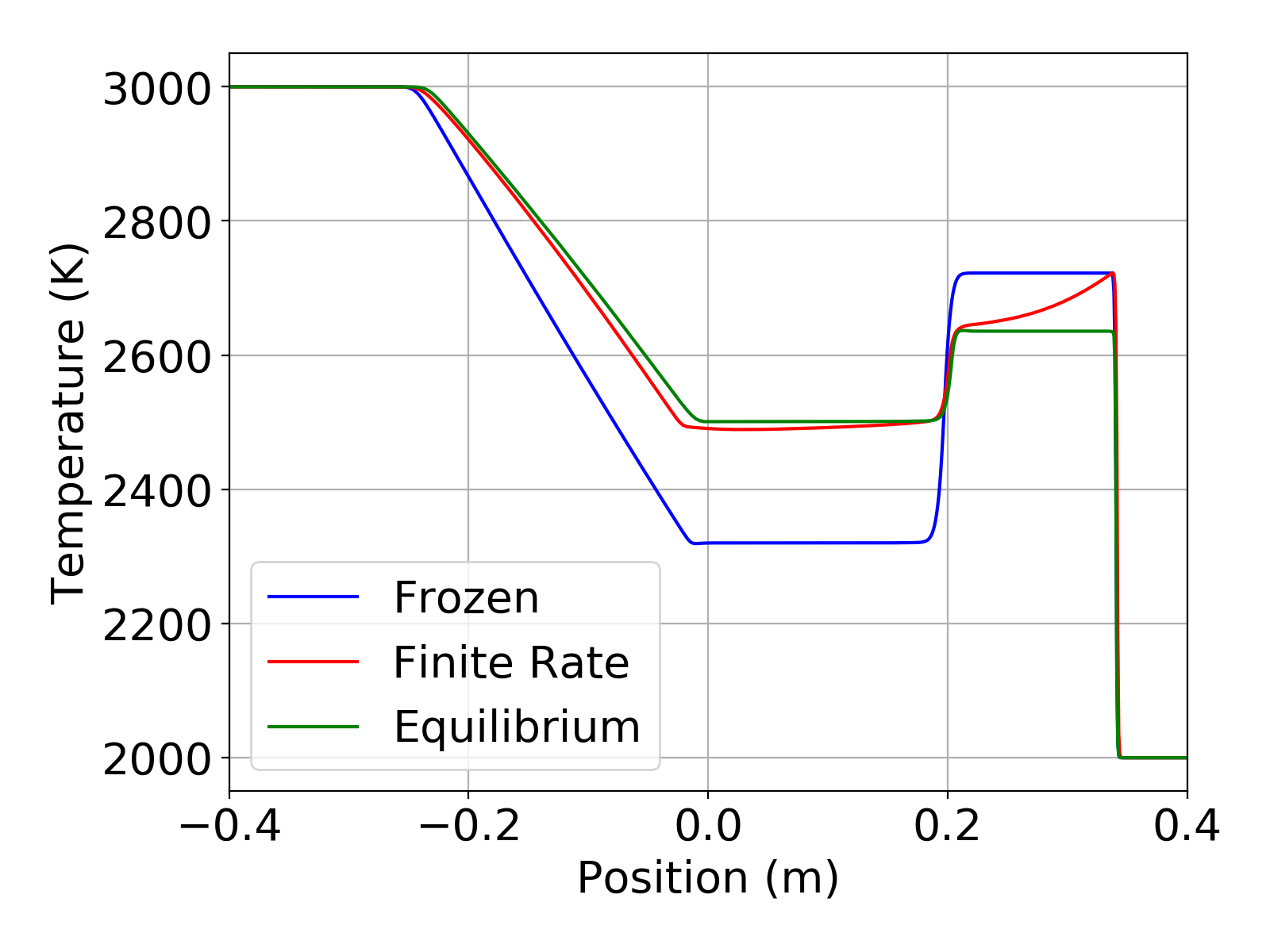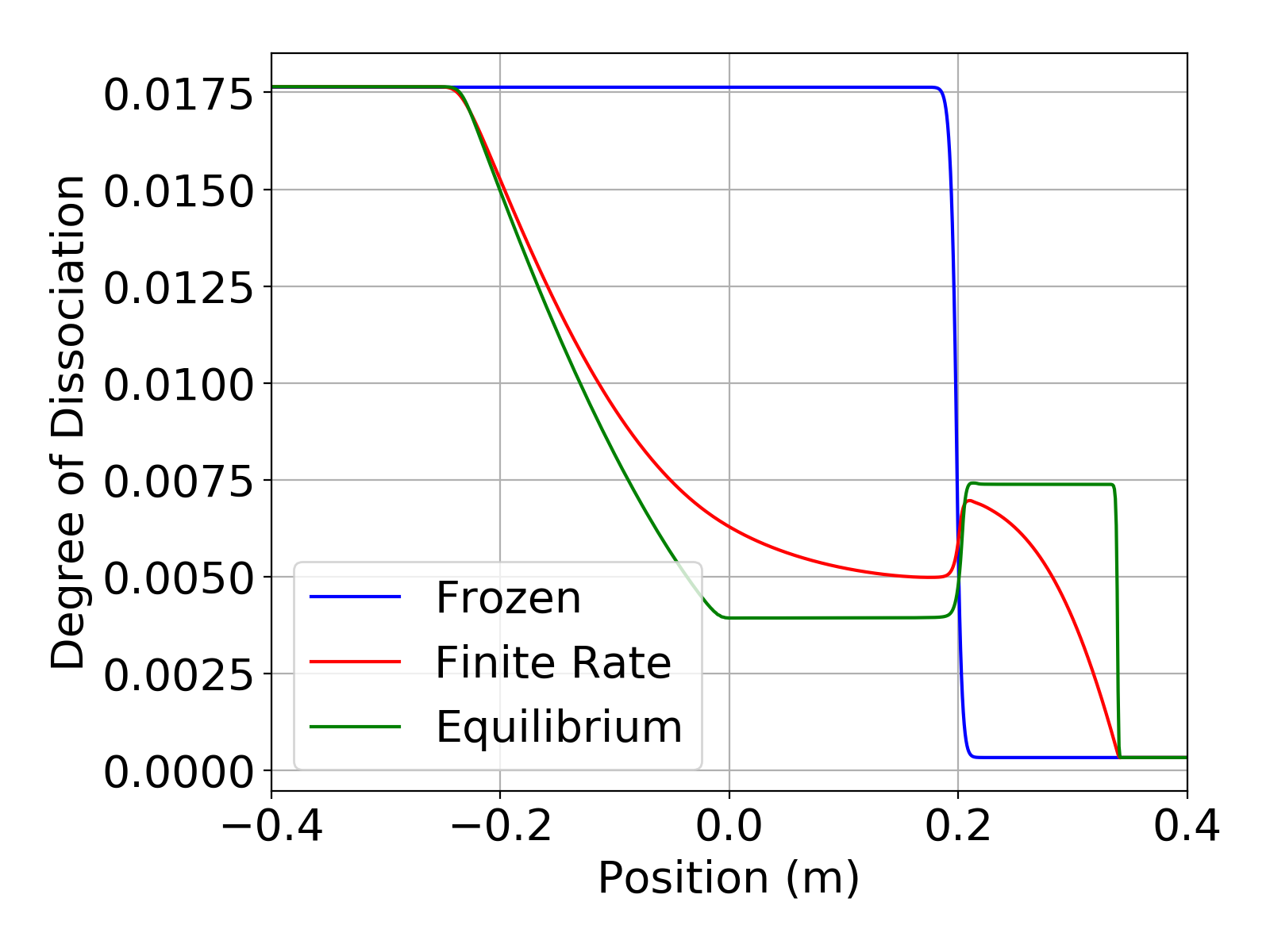## Problem Description

Version v0.9.0 of Aither added a finite rate reacting flow capability. To test this capability and to compare the effect of finite-rate chemistry to frozen chemistry and equilibrium chemistry, a test case for dissociating $O_2$ in a shock tube was added to the Aither testCases/dissociation directory. The simulation follows the description in Luke .

### Initial Conditions

The shock tube is initialized with two separate flow states. The left side is initialized with a high temperature, high pressure, equilibrium mixture of $O_2$ and $O$. Within this state there is already a significant amount of dissociation. The right state is intialized with a low temperature, low pressure, equilibrium mixture. This state has comparatively minimal dissociation. The flow states are shown below.

left: pressure=1e6 Pa, temperature=3000 K, mass fractions=[O2=0.9824, O=0.0176]
right: pressure=1e5 Pa, temperature=2000 K, mass fractions=[O2=0.9997, O=0.0003]


### Chemistry Mechanisms

The shock tube simulation was run with three separate chemistry mechanisms for comparison. A frozen chemistry model, a finite-rate model, and an equilibrium model were used. Each of these models are present in Aither as of v0.9.0.

#### Frozen Chemistry

For the frozen chemistry simulation, no reactions were present. This is the default chemistry model for Aither. In can be explicitly selected by setting the chemistryModel to none or frozen in the input file as shown below.

chemistryModel: none


## Results

The simulations were run in a time accurate manner using a time step of 2e-7 seconds for a total time of 2.4e-4 seconds. The plots below show the temperature and degree of dissociation in the shock tube at the end of the simulation. For this simple mechanism the degree of dissociation is just the mass fraction of $O$ present. The temperature plot clearly shows the effect of finite-rate chemistry in the region behind the shock. The plots also show that the finite-rate solution is bounded by the frozen and equilibrium solutions. These results from Aither show excellent agreement with those in Luke .Temperature in shock tube for frozen, finite-rate, and equilibrium chemistry mechansims.Degree of dissociation in shock tube for frozen, finite-rate, and equilibrium chemistry mechansims.

 Luke, E. A. “A Rule-Based Specification System for Computational Fluid Dynamics”. Ph. D. Thesis. 1999.